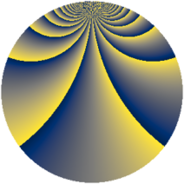# Properties

 Label 2001.2.boLevel $2001$ Weight $2$ Character orbit 2001.bo Rep. character $\chi_{2001}(4,\cdot)$ Character field $\Q(\zeta_{154})$ Dimension $7200$ Sturm bound $480$

# Related objects

## Defining parameters

 Level: $$N$$ $$=$$ $$2001 = 3 \cdot 23 \cdot 29$$ Weight: $$k$$ $$=$$ $$2$$ Character orbit: $$[\chi]$$ $$=$$ 2001.bo (of order $$154$$ and degree $$60$$) Character conductor: $$\operatorname{cond}(\chi)$$ $$=$$ $$667$$ Character field: $$\Q(\zeta_{154})$$ Sturm bound: $$480$$

## Dimensions

The following table gives the dimensions of various subspaces of $$M_{2}(2001, [\chi])$$.

Total New Old
Modular forms 14640 7200 7440
Cusp forms 14160 7200 6960
Eisenstein series 480 0 480

## Trace form

 $$7200q - 112q^{4} - 8q^{7} - 120q^{9} + O(q^{10})$$ $$7200q - 112q^{4} - 8q^{7} - 120q^{9} + 56q^{11} + 20q^{13} - 52q^{16} - 52q^{22} + 70q^{23} + 292q^{25} - 112q^{28} - 50q^{29} + 80q^{30} - 28q^{31} + 28q^{32} + 16q^{33} - 16q^{34} + 32q^{35} + 156q^{36} - 28q^{37} - 228q^{38} - 8q^{42} + 28q^{43} - 28q^{44} - 56q^{47} - 112q^{48} + 88q^{49} + 112q^{50} + 196q^{51} + 132q^{52} + 16q^{53} - 252q^{55} + 196q^{56} - 48q^{57} + 46q^{58} - 32q^{59} - 56q^{61} + 180q^{62} + 8q^{63} + 8q^{64} + 16q^{65} - 112q^{66} - 108q^{67} - 28q^{69} + 76q^{71} - 28q^{72} + 98q^{74} - 112q^{76} - 16q^{78} + 28q^{79} + 348q^{80} + 120q^{81} - 24q^{82} + 152q^{83} - 28q^{85} - 1152q^{86} + 88q^{87} - 184q^{88} + 28q^{89} - 340q^{91} + 58q^{92} + 32q^{93} - 48q^{94} - 28q^{97} - 140q^{98} + O(q^{100})$$

## Decomposition of $$S_{2}^{\mathrm{new}}(2001, [\chi])$$ into newform subspaces

The newforms in this space have not yet been added to the LMFDB.

## Decomposition of $$S_{2}^{\mathrm{old}}(2001, [\chi])$$ into lower level spaces

$$S_{2}^{\mathrm{old}}(2001, [\chi]) \cong$$ $$S_{2}^{\mathrm{new}}(667, [\chi])$$$$^{\oplus 2}$$